# Anatomy of Table Addition

With the following examples we want to demonstrate the practicality and versatility of table functions related with various addition operations.

These examples may also provide our readers with a general idea about the logic of table functions included in our .net libraries finaquant® protos (non-commercial) and finaquant® calcs (commercial).

Let’s begin with the simplest case.

### How to add a scalar number to all key figures of a table

```using FinaquantCalcs; ... ResultTable = CostTable + 500; MatrixTable.View_MatrixTable(ResultTable, "CostTable + 500");```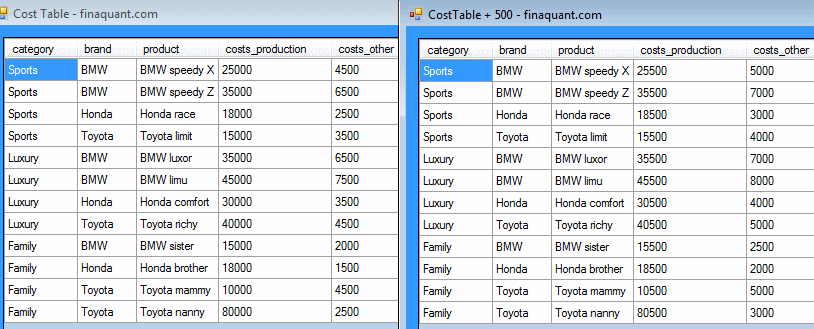What if you want to add a scalar not to all key figures, but only to a selected one?

### How to add a scalar number to a selected key figure of table

```ResultTable = MatrixTable.AddScalarToSelectedKeyFigure(CostTable, 250, InputKeyFig: "costs_other", OutputKeyFig: "costs_other"); MatrixTable.View_MatrixTable(ResultTable, "CostTable: OtherCosts = OtherCosts + 250");```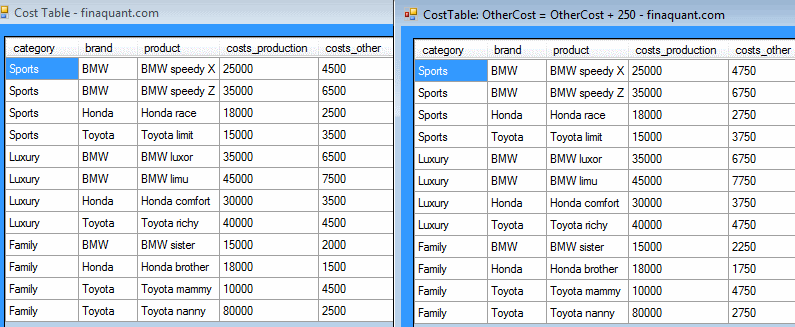Note that the resultant (output) key figure is also the input key figure in this example, namely costs_other. What if you want to see the result of the addition in another key figure?

### How to add a scalar number to a selected key figure of table with result in another key figure

```ResultTable = MatrixTable.AddScalarToSelectedKeyFigure(CostTable, 330, InputKeyFig: "costs_other", OutputKeyFig: "costs_total"); MatrixTable.View_MatrixTable(ResultTable, "CostTable: TotalCosts = OtherCosts + 330");```

Note that the new key figure costs_total which is added into the table must already be defined in meta data. Otherwise the table function above will return an error.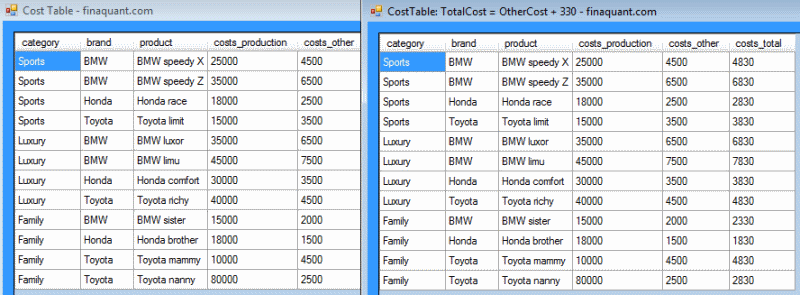So far, we were adding only a single scalar number to tables. What if you want to add key figures of a table to corresponding key figures of another table?

For example, assume you want to adjust ProductionCosts and OtherCosts with an adjustment table where these adjustments are defined per category:

### How to add up common key figures of two tables

```ResultTable = MatrixTable.AddAllKeyFigures(tbl1: CostTable, tbl2: AdjTable1); MatrixTable.View_MatrixTable(ResultTable, "ResultTable = CostTable + AdjTable1");```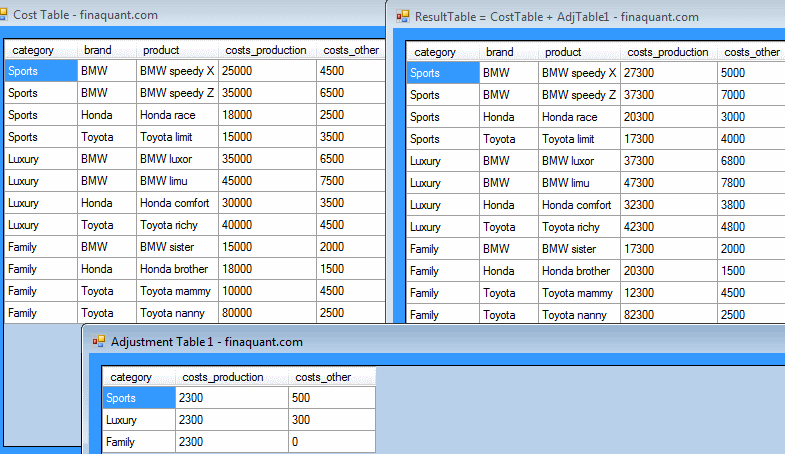Same table function could be written with the practical short notation as follows:

`ResultTable = CostTable + AdjTable1;`

Note that the adjustment table (second table in addition) need not have all the key figures of the cost table. The only condition for this table function is, first table must contain all the fields of second table.

```ResultTable = MatrixTable.AddAllKeyFigures(tbl1: CostTable, tbl2: AdjTable2); MatrixTable.View_MatrixTable(ResultTable, "ResultTable = CostTable + AdjTable2");```The tables to be added could have any number of attributes and key figures; the code and parameters of the table function wouldn’t change. Matching the attribute combinations of two tables before applying any algebraic operations is the built-in logic in most of the table functions.

In our last example we use match-all values (joker values) in the second table (addend) for attributes. Additive cost adjustments are given either per category (like sports), or per brand (like Toyota), or per category and brand (like Luxury/Honda).

### Using match-all attribute values (joker values) for table addition

```ResultTable = MatrixTable.AddSelectedKeyFigures(tbl1: CostTable, tbl2: AdjTable3, InputKeyFigTbl1: "costs_other", InputKeyFigTbl2: "costs_adjustment", OutputKeyFig: "costs_other", JokerMatchesAllvalues: true, TextJoker: "ALL", NumJoker: 0); MatrixTable.View_MatrixTable(ResultTable, "CostTable: OtherCosts = OtherCosts + Adjustments");```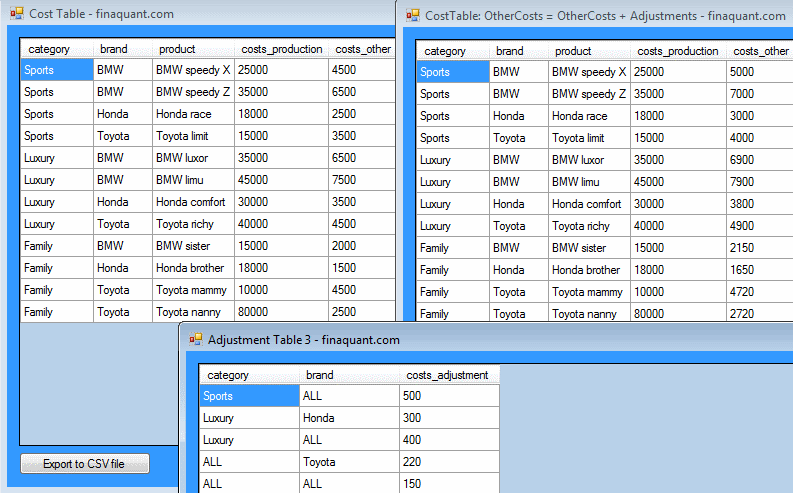### How about other algebraic operations like multiplication, division and subtraction?

Be assured; the general logic is just the same. There is a set of table functions for each algebraic operation, and more.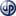Copyright secured by Digiprove © 2013 Tunc Ali Kütükcüoglu
This entry was posted in Calculation engine and tagged . Bookmark the permalink.